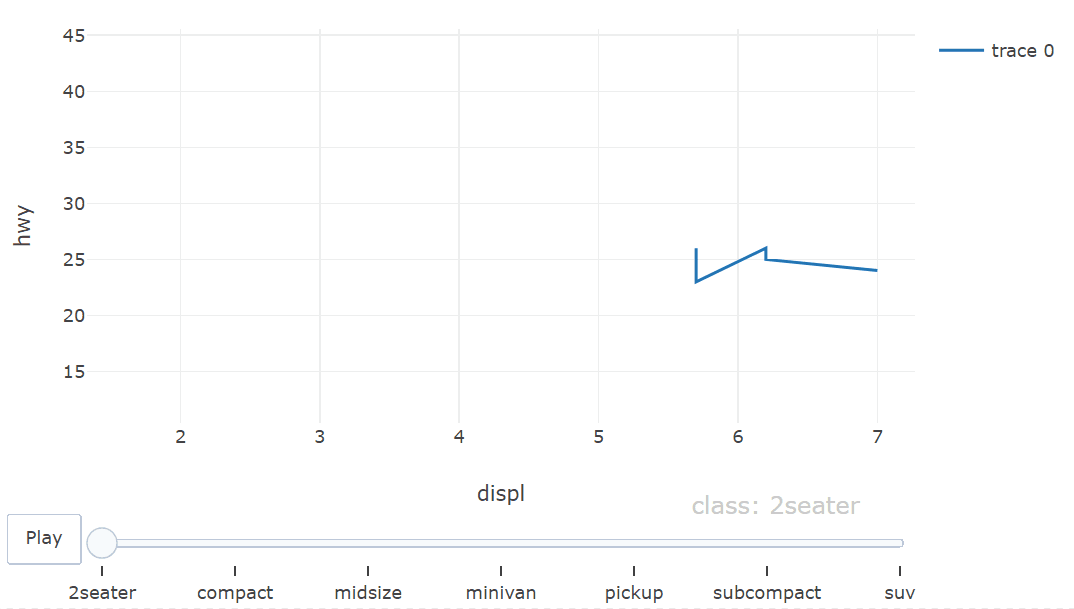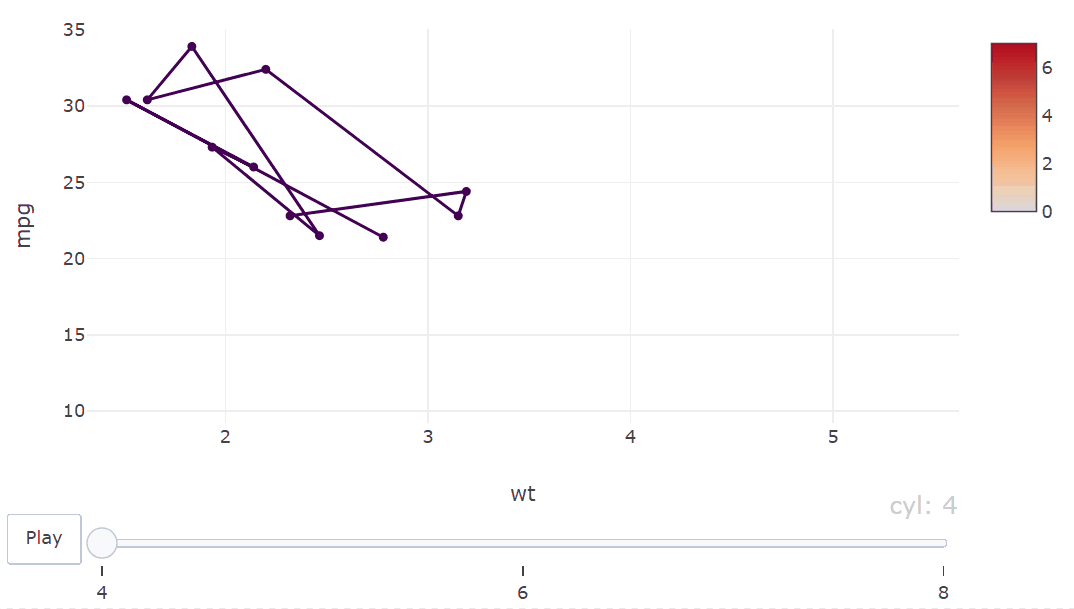GFG App
Open AppBrowser
Continue

# How to Create an Animated Line Graph using Plotly

An animated line graph is a visual representation of data that changes over time or over a categorical variable. It can be a powerful tool for visualizing trends and patterns in data and can help to communicate complex ideas in a clear and concise way. In this tutorial, we will learn how to create an animated line graph using the Plotly library in R Programming Language.

Before we dive into the steps for creating an animated line graph, it’s important to understand some of the key concepts and terminology related to this type of visualization.

• Frame: In an animated line graph, each frame represents a different point in time or a different category. When the frame changes, the data points on the graph are updated to reflect the new data.
• Animation Attributes: The animation attributes are the settings that control how the animation behaves. For example, you can specify the duration of each frame, the easing function used to transition between frames, and whether to start the animation from the current frame or from the beginning.

Now that we’ve covered the basic concepts, let’s take a look at the steps needed to create an animated line graph using Plotly in R. Load the required libraries: To use the Plotly library in R, you will need to install it first and then load it.

## R

 `# Load the Plotly and data.table libraries ` `library``(plotly) ` `library``(data.table)`

Before you can create an animated line graph, you will need to have some data to visualize. You can use your own data, or you can load one of the built-in datasets available in R.

## R

 `# Load the mpg dataset ` `data``(mpg)`

Convert the data to a data table: Plotly works best with data stored in a data table format. To convert your data to a data table, you can use the as.data.table() function. For example dt <- as.data.table(mtcars).

## R

 `# Convert the mpg data to a data table ` `dt <- ``as.data.table``(mpg)`

To create a Plotly line graph, you can use the plot_ly() function. You will need to specify the x and y variables, as well as the type and mode of the graph.

## R

 `# Create a Plotly line graph ` `p <- ``plot_ly``(dt, x = ~displ, ` `             ``y = ~hwy, ` `             ``type = ``"scatter"``, ` `             ``mode = ``"lines"``, ` `             ``frame = ~class) `

To make the line graph animated, you will need to add the animation_frame and animation_opts attributes to the graph. The animation_frame attribute specifies which column to use as the animation frame, and the animation_opts attribute contains a list of options that control the behavior of the animation.

## R

 `# Add the animation attributes ` `p <- ``layout``(p, ` `            ``animation_opts =  ` `            ``list``(frame = ``list``(duration = 500, ` `                              ``redraw = ``TRUE``))) ` ` `  `# Display the graph ` `p`

Output:Animated Line Graph using Plotly

Example: In this example, we’ll create a line graph that animates the data points over a categorical variable, with custom colors for each frame. We’ll use the mtcars dataset.

## R

 `# Load the Plotly and data.table libraries ` `library``(plotly) ` `library``(data.table) ` ` `  `# Load the mtcars dataset ` `data``(mtcars) ` ` `  `# Convert the mtcars data to a data table ` `dt <- ``as.data.table``(mtcars) ` ` `  `# Set custom colors for each frame ` `colors <- ``c``(``"#FF0000"``, ``"#00FF00"``, ``"#0000FF"``) ` ` `  `# Create a Plotly line graph ` `p <- ``plot_ly``(dt, x = ~wt, y = ~mpg, ` `             ``type = ``"scatter"``, ` `             ``mode = ``"lines"``, ` `             ``frame = ~cyl, ` `             ``color = ~cyl) %>% ` `  ``style``(marker = ``list``(color = ~cyl, ` `                      ``colors = colors)) ` ` `  `# Add the animation attributes ` `p <- ``layout``(p, ` `            ``animation_opts =  ` `            ``list``(frame = ``list``(duration = 5000, ` `                              ``redraw = ``TRUE``))) ` ` `  `# Display the graph ` `p`

Output:Animated Line Graph using Plotly with color changes

My Personal Notes arrow_drop_up